# JAC Class 9 Science Important Questions Chapter 12 Sound

## JAC Board Class 9th Science Important Questions Chapter 12 Sound

Multiple Choice Questions

Question 1.
Which part of the human ear converts sound vibrations into electrical signals?
(a) Malleus
(b) Incus
(c) Tympanic membrane
(d) Cochlea
(d) Cochlea

Question 2.
What do dolphins, bats, and tortoises use to hear the sound?
(a) Ultrasound
(b) Infrasound
(c) Both (a) and (b)
(d) None of these
(a) Ultrasound

Question 3.
Children under the age of 5 can hear upto
(a) 25 Hz
(b) 25 kHz
(c) 20 Hz
(d) 20 kHz
(b) 25 kHzQuestion 4.
Multiple reflections of sound are used in
(a) stethoscope
(b) trumpet
(c) megaphone
(d) all of these
(d) all of these

Question 5.
To hear a distinct echo, the time interval between the original sound and the reflected sound must be at least
(a) 0.2s
(b) 1s
(c) 2s
(d) 0.1s
(d) 0.1s

Question 6.
Speed (v), wavelength (l) and the frequency (v) of a sound wave are related as
(a) λ = v × λ
(b) v = λ – v
(c) v = λ × v
(d) v = λ/v
(c) v = λ × v

Question 7.
Speed of sound depends upon
(a) temperature of the medium
(b) density of the medium
(c) temperature of source producing sound
(d) temperature and density of the medium
(d) temperature and density of the medium

Question 8.
Using which characteristic of sound can we distinguish between the sounds having same pitch and loudness?
(a) Tone
(b) Note
(c) Pitch
(d) Timber
(d) Timber

Question 9.
Loud sound can travel a larger distance, due to
(a) higher amplitude
(b) higher energy
(c) higher frequency
(d) higher speed
(a) higher amplitude

Question 10.
A wave in slinky travelled to and fro in 5 s. The length of the slinky is 5 m. What is the velocity of the wave?
(a) 10 m/s
(b) 5 m/s
(c) 2 m/s
(d) 25 m/s
(b) 5 m/s

Question 11.
The reciprocal of frequency is
(a) amplitude
(b) wavelength
(c) time – period
(d) wave velocity
(c) time – period

Question 12.
To and fro motion of an object is called
(a) wave
(b) vibrations
(c) amplitude
(d) all of the above
(b) vibrations

Question 13.
Which of the following frequencies will be audible to the human ear?
(a) 5 Hz
(b) 5000 Hz
(c) 27000 Hz
(d) 50000 Hz
(b) 5000 Hz

Question 14.
If the distance between a crest and its consecutive trough is L, then the wavelength of the wave is given by
(a) L
(b) 2L
(c) L/2
(d) 4L
(b) 2L

Question 15.
What is the nature of the ocean waves in deep water?
(a) Transverse
(b) Longitudinal
(c) Both transverse and longitudinal
(d) None of these
(c) Both transverse and longitudinal

Analysing & Evaluating Questions

Question 16.
A key of a mechanical piano is struck gently and then struck again but much harder this time. In the second case
(a) sound will be louder but pitch will not be different
(b) sound will be louder and pitch will also be higher
(c) sound will be louder but pitch will be lower
(d) both loudness and pitch will remain unaffected
(a) sound will be louder but pitch will not be differentQuestion 17.
Which kind of sound is produced by an earthquake before the main shock wave begins?
(a) ultrasound
(b) infrasound
(c) audible sound
(d) none of these
(b) infrasound

Question 18.
Before playing the orchestra in a musical concert, a sitarist tries to adjust the tension and pluck the string suitably. By doing so, he is adjusting
(a) intensity of sound only
(b) amplitude of sound only
(c) frequency of the sitar string with the frequency of other musical instruments
(d) loudness of sound
(c) frequency of the sitar string with the frequency of other musical instruments

Assertion Reason Questions

Directions: In the following questions, the Assertions and the Reasons have been put forward. Read the statements carefully and choose the correct alternative from the following:
(A) Both the assertion and the reason are correct and the reason is the correct explanation of the assertion.
(B) The assertion and the reason are correct but the reason is not the correct explanation of the assertion.
(C) The assertion is true but the reason is false.
(D) Both the statements are false.
1. Assertion:
Sound travels as a longitudinal wave in air.
Reason: Sound wave needs a medium for its propagation.
(B) The assertion and the reason are correct but the reason is not the correct explanation of the assertion.

2. Assertion: Speed of sound increases on increasing the temperature of the medium through which its propagates.
Reason: On increasing the temperature, kinetic energy of the particles of medium increases.
(B) The assertion and the reason are correct but the reason is not the correct explanation of the assertion.

3. Assertion: Stage of an auditorium has a curved sound board behind the stage.
Reason: Curved wall spreads sound in all directions evenly.
(A) Both the assertion and the reason are correct and the reason is the correct explanation of the assertion.

4. Assertion: Sound travels faster in solids than in liquids.
Reason: Particles of solids are closely packed as compared to those of liquids.
(A) Both the assertion and the reason are correct and the reason is the correct explanation of the assertion.

5. Assertion: Pitch of a sound wave depends on its frequency.
Reason: Higher the frequency, lesser is the pitch of the sound wave.
(C) The assertion is true but the reason is false.

Question 1.
What is SONAR?
SONAR (Sound Navigation And Ranging) is a technique used for determining water depth and locating underwater objects, such as reefs, submarines and school of fish.

Question 2.
Define one hertz.
One hertz is one vibration per second.

Question 3.
Name the two types of mechanical waves.
The two types of mechanical waves are:

1. Transverse wave
2. Longitudinal wave

Question 4.
What is a wave?
A wave is a disturbance that travels in a medium due to repeated periodic motion of particles about their mean position, such that the disturbance is handed over from one particle to the other without the actual movement of the particles of the medium.

Question 5.
What is a longitudinal wave?
It is a wave in which the particles of the medium vibrate in the direction of propagation of the wave.

Question 6.
What is a transverse wave?
It is a wave in which the particles of the medium vibrate perpendicular to the direction of propagation of the wave.

Question 7.
What do you understand by the term ‘echo’?
The sound heard after reflection from a rigid obstacle back to the listener is called ‘echo’.

Question 8.
What is ‘pitch’?
The way our brain interprets the frequency of an emitted sound is called ‘pitch’.

Question 9.
What is ‘note’ of a sound?
The sound produced due to a mixture of several frequencies is called a ‘note’.

Question 10.
What are ‘ultrasonic’ and ‘infrasonic’ sound waves?
Sound waves with frequencies below the audible range (less than 20 Hz) are termed as ‘infrasonic sound waves’ and sound waves with frequencies above the audible range (more than 20,000 Hz) are termed as ‘ultrasonic sound waves’.

Analysing & Evaluating Questions

Question 11.
The speed of sound in vulcanised rubber is much lower than in the common solids. Give a proper explanation.
Rubber is soft, porous and sound absorber.

Question 12.
A sound wave corresponds to larger number of compressions and rarefaction passing through a fixed point per unit of time. Should it have higher pitch or lower pitch?
Such a sound wave will have higher pitch, (i.e., higher frequency).

Question 13.
An echo is heard on a day when temperature is about 22°C. Will the echo be heard sooner or later if the temperature falls to 4°C?
At lower temperature, the speed of sound will decrease. Therefore, to travel through the same distance, it will take more time. So the echo will be heard later.

Question 1.
What are mechanical or elastic waves? Give examples.
The waves which require a material medium for their propagation are called f mechanical waves. They are also called elastic waves because their propagation depends on the elastic properties of the medium.

Examples of mechanical waves:
(a) Sound waves in air.
(b) Waves over water surface.
(c) Waves produced during earthquake (seismic waves).Question 2.
What are electromagnetic waves? Cite examples.
The waves which do not require a material medium for their propagation are called electromagnetic waves. Such waves travel through vacuum with a speed of 3 × 108 m/s.
Examples of electromagnetic waves:
(a) Light waves
(b) X – rays
(d) Microwaves

Question 3.
Define ‘crests’ and ‘troughs’ of a wave.
In transverse waves, the particles of the medium which have maximum displacement in the positive Y – direction are called ‘crests’ while those which have maximum displacement in the negative Y – direction are called ‘troughs’.

Question 4.
Define the terms ‘compressions’ and ‘rarefactions’.
When longitudinal waves pass through a medium, these cause pressure variations in different parts of the medium. The regions of increased pressure are called ‘compressions’ and the regions of decreased pressure are called ‘rarefactions’.

Question 5.
Differentiate between sound and light waves.

 Sound wave Light wave (a) Travels in the form of longitudinal wave. (a) Travels in the form of transverse wave. (b) Requires a medium for its propagation. (b) Does not require a medium for its propa – gation. (c) Travels through air with a speed of 332 m/s at 0°C. (c) Travels through air with a speed of nearly 3 × 108 m/s.

Question 6.
Why are the longitudinal waves also called pressure waves?
Longitudinal waves travel in a medium as a series of alternate compressions and rarefactions, i.e., as they travel, there is a variation in pressure across the medium. Therefore, they are called pressure waves.

Question 7.
Derive a relation between wavelength, frequency and velocity of a wave.
Frequency, wavelength and wave velocity are related as follows: Wavelength is the distance travelled by the wave during the time a particle of the medium takes to complete one vibration. Therefore, if λ be the wavelength and T be the time – period, the wave travels a distance λ in time T.
Hence, Wave velocity = $$\frac{Distance}{Time}$$
⇒ v = $$\frac{\lambda}{\mathrm{T}}$$
⇒ v = λ v
∵ {$$\frac{1}{T}$$ = frequency (v)}
∴ Wave velocity = Frequency × Wavelength
The wave velocity in a medium remains constant under the same physical conditions.

Question 8.
On what factor does the frequency of a wave depend?
Frequency of a wave is given by,
v = $$\frac{\mathrm{v}}{\lambda}$$
Where,
v is the speed with which the wave propagates, and λ is the wavelength of the wave. Different sources produce oscillations of different frequencies depending on the wavelength of the sources. Frequency changes such that the speed remains constant.

Question 9.
A boat at anchor is rocked by waves whose consecutive crests are 100 m apart. The wave velocity of the mocking crests is 20 m/s. What is the frequency of rocking of the boat?
Wavelength, λ = Distance between two successive crests = 100 m
Velocity, v = 20 m/s
Frequency, v = $$\frac{\mathrm{v}}{\lambda}$$ = $$\frac{20}{100}$$ = 0.20 Hz.

Question 10.
Why do the animals behave in apeculiar manner before an earthquake?
When the earthquake strikes, it produces low frequency infrasounds before the main shock waves. These infrasonic waves are not audible to the human ears. But animals are able to detect these waves and hence some animals get disturbed before earthquakes and start behaving in a different manner.Question 11.
Differentiate between longitudinal wave and transverse wave.

 Longitudinal wave Transverse wave (a) It needs a medium for propagation. (a) It may or may not need a medium for propagation. (b) Particles of the medium move in a direction parallel to the direction of propagation of the disturbance. (b) Particles of the medium move in a direction perpendicular to the direction of propagation of the disturbance. Example: Sound wave. Examples: Light wave and seismic wave.

Question 12.
What are multiple echoes? Give examples.
The successive reflection of a sound wave from a number of obstacles results in hearing the echo of the sound transmitted one after the other. When a sound is repeatedly reflected between two parallel distant buildings or cliffs, multiple echoes are produced. For example, rolling of thunder is due to successive reflections between clouds and land surfaces.

Question 13.
A sound wave causes the density of air at a place to oscillate 1200 times in 2 minutes. Find the time period and frequency of the wave.
Frequency = $$\frac{1200}{2 \times 60}$$ = 10 Hz.
Time period = ?
Frequency = $$\frac{1}{\mathrm{~T}}$$
T = $$\frac{1}{Frequency}$$ = $$\frac{1}{10}$$ = 0. 1s.

Analysing & Evaluating Questions

Question 14.
The speed of certain waves depends on the source and the medium through which they travel.
1. What kind of waves are these?
2. Give one example of the answer to (a).

1. These waves are mechanical waves.
2. Sound waves, water waves, string waves are mechanical waves.

Question 15.
The sound of an explosion on the surface of a lake is heard by a boatman 100 m away and by a diver 100 m below the point of explosion.
1. Who will hear the sound of explosion first?
2. If sound takes time t seconds to reach the boatman, how much time (approximately) will it take to reach the diver?
1. The diver who is 100 m below the point of explosion will hear the sound of explosion first because sound travels much faster in water than in air.

2. Time taken by sound to reach the diver
= $$\frac{ Speed of sound in air × t}{Speed of sound in Water }$$
= $$\frac{344 \mathrm{~m} \mathrm{~s}^{-1}}{1533 \mathrm{~m} \mathrm{~s}^{-1}} \times \mathrm{ts}$$
= 0.22 t seconds

Question 1.
State the factors on which the speed of sound in a gaseous medium depends.
The speed of sound in a gas depends on the following factors:
(a) Effect of density: At constant pressure, the speed of sound in a gas is inversely proportional to the square root of its density.
Speed of sound ∝ = $$\frac{1}{\sqrt{\text { Density of gas }}}$$

(b) Effect of humidity: Sound travels faster in moist air than in dry air.

(c) Effect of temperature: The speed of sound in a gas is directly proportional to the square root of its absolute temperature.
Speed of sound ∝= $$\sqrt{\text { Absolute temperature of gas }}$$
So, the speed of sound increases with the increase in temperature of the gas. For example, the speed of sound in air is 331 ms-1 at 0°C and 344 ms-1 at 20°C.

(d) Effect of wind: Sound is carried by air, so the speed of sound increases when the wind blows in the direction of sound and speed of sound decreases when the wind blows in the direction opposite to the direction of sound.Question 2.
Explain some important applications of ultrasound in industry and medicine.
Applications of ultrasound in industry and medicine are as follows:

• Industrial applications:
1. The construction of big structures like buildings, bridges, machines, scientific equipment, etc., use metallic components.
2. The cracks or holes inside the block reduce the strength of the structure. Such defects are not visible from outside. Ultrasonic waves can be used to detect such defects.
3. Ultrasonic waves are allowed to pass through the metal block and detectors are used to detect the transmitted waves. If there is even a small defect, the ultrasound gets reflected back and does not reach the detector. This is how the presence of a flaw or defect is detected.
• Medical applications:
1. Echocardiography: In this technique, ultrasonic waves are made to reflect from various parts of the heart to form the image of the heart.
2. Ultrasonography: Ultrasonic waves can be used to develop three dimensional photographs of different parts of the human body. This technique is called ultrasonography.
• This technique is also used to monitor the growth of a foetus inside its mother’s womb In this technique, the ultrasonic waves travel through the tissues of the body and get reflected from a region where there is a change of tissue density.
• These waves are then converted into electrical signals that are used to generate images of the organ. These images are then displayed on a monitor or printed on a film.
3. In surgery: Ultrasonic waves are commonly used in cataract removal. Ultrasonic waves are also used to grind small stones formed in the kidneys. These grinded grains are flushed with urine.

Question 3.
State some important characteristics of wave motion.
Characteristics of wave motion are as follows:

1. It is the disturbance which travels forward through the medium and not the particles of the medium. The particles of the medium merely vibrate about their mean positions.
2. Each particle receives vibrations a little later than its preceding particle.
3. The velocity with which wave travels is different from the velocity of the particles with which they vibrate about their mean positions.
4. The wave velocity remains constant in a given medium while the particle velocity changes continuously during its vibration about the mean position.

Question 4.
How can a longitudinal wave be represented graphically?
Graphical representation of a longitudinal wave: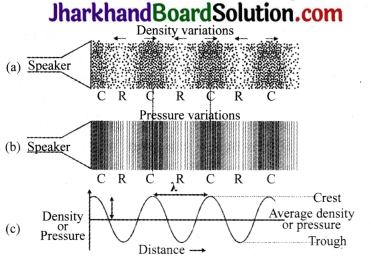Sound propagates as density or pressure variations as shown in (a), (b) and (c).

1. When a longitudinal wave passes through a medium, the particles of the medium come closer together and move away from one another alternately. Thus, alternate regions of increased and decreased density are created. These regions are called compressions and rarefactions respectively.
2. The figures (a) and (b) represent the density and pressure variations, respectively, as the sound wave propagates through a medium.
Figure (c) represents the variations of density and pressure graphically.
3. The variation of density increases or decreases as the pressure of the medium at a given time increases or decreases with distance, above and below the average value of density and pressure.
4. The distance between two successive compressions (C) or two successive rarefactions (R) is called wavelength. It is usually represented by λ (lambda).

Question 5.
Explain the formation of waves with the help of an example.
A wave is a pattern of disturbance which travels through a medium due to repeated vibrations of the particles of the medium, the disturbance being handed over from one particle to the next. The motion of the disturbance is called wave motion. If we drop a pebble into a pond of still water, a circular pattern of alternate crests and troughs spreads out from the point where the pebble strikes the water surface. The kinetic energy of the pebble makes the particles which come in contact with it oscillate.

These particles, in turn, transfer energy to the particles of next layer which begin to oscillate. Energy is further transferred to the particles of the next layer which also begin to oscillate and so on. In this way, energy is carried by the waves from one part to another. Further, if we throw pieces of paper or a cork on the water surface, it will oscillate up and down about its mean position and will not move forward with the wave. This shows that it is the disturbance of the wave which travels forward and not the particles of the medium.

Question 6.
A ship sends out ultrasound that returns from the seabed and is detected after 4 s. If the speed of ultrasound through seawater is 1550 m/s, what is the distance of the seabed from the ship?
Time between transmission and detection, t = 4s
Speed of ultrasound in sea water,
v = 1550 m/s
Distance travelled by the ultrasound
= 2 × depth of the sea = 2d
where d is the depth of the sea.
2d = Speed of sound × time = 1550 m/s × 4s = 6200m
d =$$\frac{6200}{2}$$ m = 3100 m = 3.1 km.
Thus, the distance of the seabed from the ship is 3100 m or 3.1 km.

Analysing & Evaluating Questions

Question 7.
A sound were travelling in a medium is represented as shown in the figure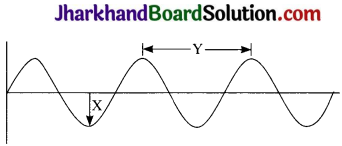(a) Which letter represents the amplitude of the sound wave?
(b) Which letter represents the wavelength of wave?
(c) What is the frequency of the source of sound if the vibrating source of sound makes 360 oscillations in 2 minutes?
In the figure,
(a) The amplitude is represented by X.
(b) The wavelength is represented by Y.
(c) Frequency
= $$\frac{No. of oscillations }{ Time in sec onds}$$ = $$\frac{360}{2 \mathrm{~min}}$$
= $$\frac{360}{2 \times 60 \mathrm{~s}}$$ = 3s-1 = Hz

Activity-1

• Take a tuning fork and set it vibrating by striking its prong on a rubber pad. Bring it near your ear and observe.
• Touch one of the prongs of the vibrating tuning fork with your finger and see what happens.
• Take a table tennis ball or a small plastic ball Now,Take a big needle and a thread, put a knot at one end of the thread, and then with the help of the needle, pass the thread through the ball. Suspend the ball from a support. Now, touch the ball gently with the prong of a vibrating tuning fork. Observe what happens.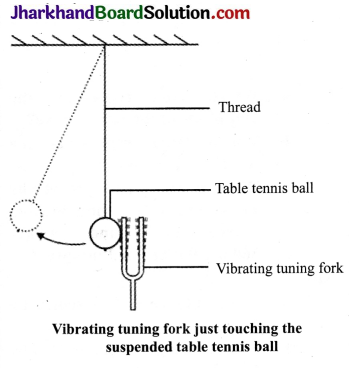Observations

• A sound is heard on bringing the vibrating fork near the ear.
• If we touch the ball with a turning fork set into vibration, the ball gets displaced from its mean position and starts oscillating.

Activity 2

• Fill water in a beaker or a glass up to the brim. Gently touch the water surface with one of the prongs of the vibrating tuning fork, as shown in the figure.
• Next, dip the prongs of the vibrating tuning fork in water, as shown in the figure.
• Observe what happens in both the cases.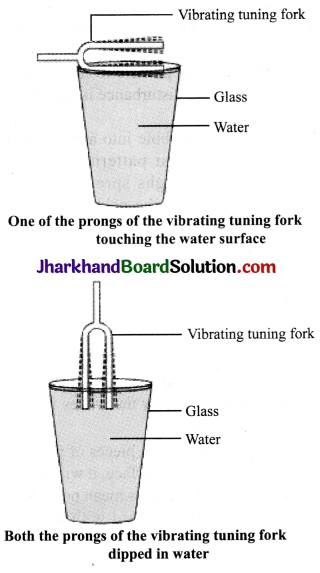Observations
In both the cases, sound will be produced by the turning fork which produces ripples in water. But in case (1), ripples are produced which will move up and down and in case (2), ripples are produced which will move sideways.

Activity 3

• Take a spring. Ask your friend to hold one of its end. You hold the other end. Now stretch the slinky as shown in the figure. Then give it a sharp push towards your friend and observe.
• Move your hand pushing and pulling the slinky alternatively and observe again.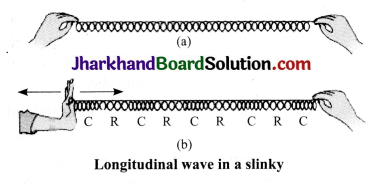Observations

• When we give a small jerk, a hump is produced and this travels forward. It represents a transverse wave.
• When we give a sharp push, a continuous disturbance is produced. This disturbance starts moving in the forward and backward direction parallel to the direction of propagation of the disturbance. It represents a longitudinal wave.
• If you mark a dot on the slinky, you will observe that the dot on the slinky will move back and forth parallel to the direction of the propagation of the disturbance.

Activity 4

• Take two identical pipes, as shown in the figure. You can make pipes using a chart paper. The length of the pipes should be sufficiently long as shown.
• Arrange them on a table near a wall.
• Keep a clock near the open end of one of the pipes and try to hear the sound of the clock through the other pipe.
• Adjust the position of the pipes, so that you can hear the best sound of the clock.
• Now, measure the angles of incidence and reflection and see the relationship between the angles.
• Lift the pipe on the right, vertically to a small height and observe what happens.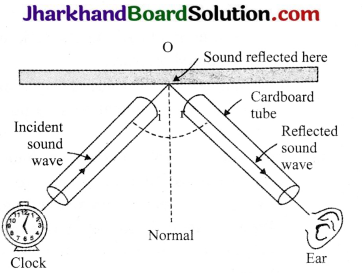Observations

• Reflection of sound is similar to the reflection of light, i.e., angle of incidence = angle of reflection.
• If we lift the pipe vertically to a small height, we will not be able to hear the sound through the other end of the pipe because the incident sound wave, the reflected sound wave and the normal, all he in the same plane.

Value Based Questions

Question 1.
Sahil noticed that his pet dog was frightened and trying to hide in a safe place in his house when some crackers were burst in the neighbourhood. He realised the problem and he decided not to burst crackers during diwali or for any other celebrations.
1. What must be the range of sound of crackers?
2. Name two diseases that can be caused due to noise pollution.
3. Name the values of Sahil reflected in the above act.

1. The range of crackers sound must be between 20 Hz and 20 kHz.
2. Two diseases that can occur due to noise pollution are heart attack and high blood pressure.
3. Sahil reflects the value of respect and sensitivity for animals and caring for animals.

Question 2.
It is not advisable to construct houses near airports. Inspite of that, many new apartments are constructed near airports. Rahul files RTI and also complains to the municipal office about the same?
1. Why should we not reside near airport?
2. Name two other places where there is noise pollution.
3. What value of Rahul is reflected in this act?